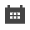# Factors that reduce the separation effect of cycloneThe impact of the structural parameters and process conditions of the cyclone on the separation effect:

1. Cone angle. As the cone angle increases, the sorting density increases, and vice versa, as the cone angle decreases, the sorting density decreases. Generally, the cone angle of the single-cone-angle aqueous medium cyclone is about 90°.

2. Three diameters of cyclone. The diameter of the feed pipe increases and the flow rate increases. It is necessary to ensure that the speed of the feed port is greater than 6-12m/s; the diameter of the overflow pipe increases, the flow rate increases, and the separation density increases. It is necessary to ensure that the material has a certain retention in the cyclone. Time; the diameter of the underflow increases, the sorting density decreases, the diameter of the underflow decreases, the sorting density increases, and the smooth discharge of the underflow material must be ensured. The ratio of the diameter of the underflow to the diameter of the overflow pipe is called the cone ratio. If the cone ratio is large, the sorting density will decrease; if the cone ratio is small, the sorting density will increase.

3. Insertion depth of overflow pipe. Usually, the vertical distance between the bottom of the overflow pipe of the cyclone and the interface between the cylinder and the cone is represented by △H, which is called the free gap. Increasing △H, the sorting density decreases; decreasing △H, sorting density increases.

4. Inlet pressure. In order to ensure sufficient centrifugal force, the pressure at the feed inlet should increase with the increase of the diameter of the cyclone and the processing capacity.

### Related Articles

Product Knowledge
Solutions
FAQ